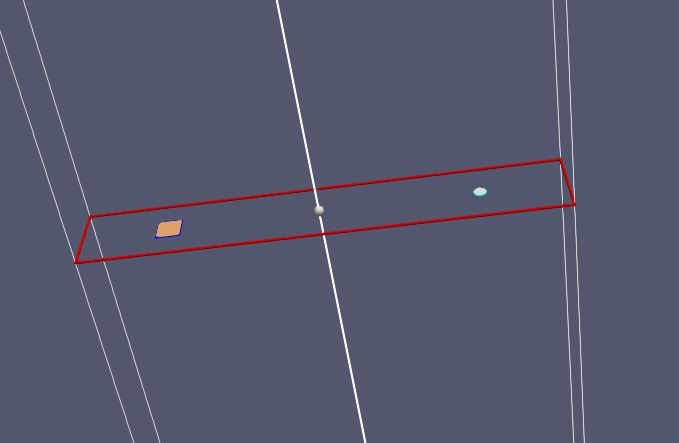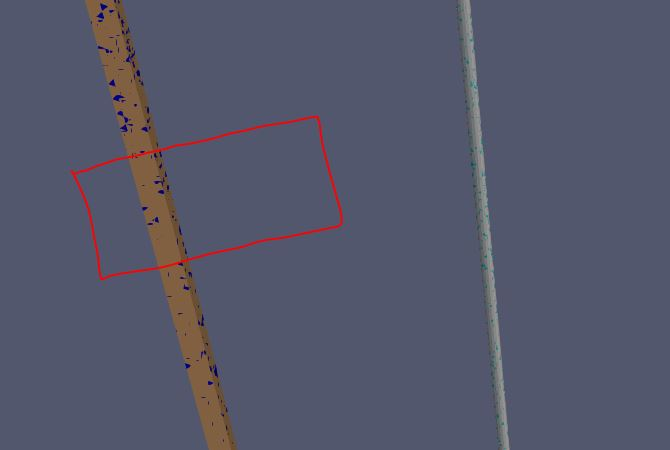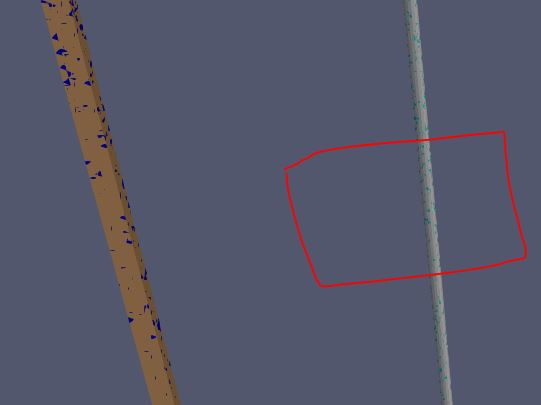# question about 'Slice filter' in paraview

Hello, i am beginner of paraview.

when i am using the slice filter to get face-averaged values(temperature, velocity etc) in 3D analysis, i had a question.

when i used the slice filter, i knew that the slicing range is same as geometry size or is larger than geometry size,

however, the slicing range can not be smaller than the geometry size,

in other words, i want to get the cross section of square tube and circular tube respectively. however, when i used this slice filter, because the slicing range can not be smaller than geometry size, i got the cross sectional area of square tube and circular tube at the same time. but, as i mentioned before, i want to obtain the cross section of square tube and circular tube “respectively”
i have to separate them!

is there anyone to help me? is there function of modifying the range of slicing of which size is smaller than geometry size?

[problem that i confront]

→ so i can only get the cross section of square tube and circular tube at the same time. i do not want this…[i want]→ so i want tn cross section of square tube and circular tube respectively, but i do not know the method to realize it

thank you very much!

I’m a bit confused by your needs, but did you try changing the axis of the slice to Z ?

Best.

i found the method!

at first, using clip, the range can be adjusted. and then, using slice, i can achieve my purpose

thank you!